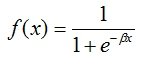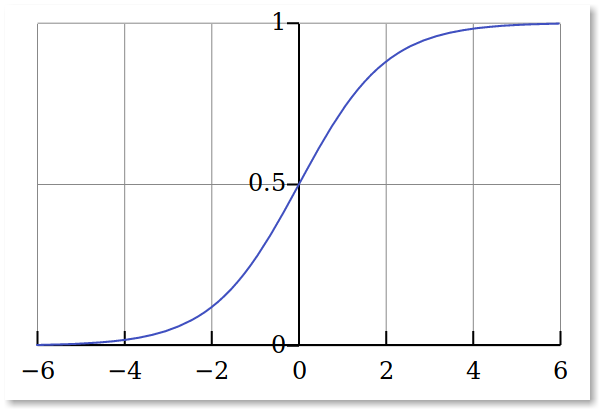Lets make a spam filter using logistic regression. We will classify messages to be either ham or spam. The dataset we’ll use is the SMSSpamCollection dataset. The dataset contains messages, which are either spam or ham.

Related course:

## what is logistic regression?

Logistic regression is a simple classification algorithm. Given an example, we try to predict the probability that it belongs to “0” class or “1” class.

Remember that with linear regression, we tried to predict the value of y(i) for x(i). Such continous output is not suited for the classification task.

Given the logisitic function and an example, it always returns a value between one and zero.Lets plot the data for that function. We’ll use the range {-6,6}:This shows an S shape. The inverse of the logistic function is called the logit function. To make the correlation between the predictor and dependent variable linear, we need to do the logit transformation of the dependent variable.

Logit = Log (p/1-p) = β 0 + β x

We can now apply it to the binary classification task.

Related course:

## spam filter code

We load the dataset using pandas. Then we split in a training and test set. We extract text features known as TF-IDF features, because we need to work with numeric vectors.

Then we create the logistic regression object and train it with the data. Finally we create a set of messages to make predictions.